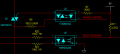# Calculating Power Dispated across triac

#### wd8cxb

Joined Jul 8, 2009
10
I have a circuit that will PWM a triac for phase control of a motor. I am really confused on how to size the heat sink for the operation of the circuit.

I have measured the voltage drop across the Triac and used the current to find the "ON" resistance but I want to be able to calculate it. I used the knee voltage chart but not sure I am calculating it correctly. My Case to Junction temp with my selected heat sink is about 140°C. My heat sink's thermal resistance is 15°C/W.

Due to size and cost I need to be able to accurately calculate the required heatsink. Once I find the power dissipated, calculating the heatsink is not a problem.

The current will be 13A and the voltage supplied to the motor 115V@50Hz.

The triac is a little fuse Q6016LH3 (Isolated Tab TO-220). http://www.littelfuse.com/~/media/electronics/datasheets/switching_thyristors/littelfuse_thyristor_qxx15xx_qxx16xhx_datasheet.pdf.pdf

Here is the circuit.Any suggestion on calculating the heat dissipation or ways to improve the circuit would be appreciated.

David

#### Lestraveled

Joined May 19, 2014
1,946
OK, the max dissipation will be when the triac is on all the time. Per the spec sheet, the MT voltage is 1.7 volts, so, 13 amps times 1.7 volts equals 22.1 watts.

#### crutschow

Joined Mar 14, 2008
25,126
OK, the max dissipation will be when the triac is on all the time. Per the spec sheet, the MT voltage is 1.7 volts, so, 13 amps times 1.7 volts equals 22.1 watts.
Actually it would be the average current times the diode drop not the RMS current but since the average of a sinewave is only about 10% less than the RMS, that's not a big difference.